# Automatic Calibration of Sociotechnical Systems Simulation Models on The Example of The Infection Spread Model

Title: Automatic Calibration of Sociotechnical Systems Simulation Models on The Example of The Infection Spread Model
Daria A. Zubkova, Valeriya V. Rakova, Zhanna V. Burlutskaya, Aleksei M. Gintciak

Corresponding email:

Zubkova, D.A., Rakova, V.V., Burlutskaya, Z.V., Gintciak, A.M., 2022. Automatic Calibration of Sociotechnical Systems Simulation Models on The Example of The Infection Spread Model . International Journal of Technology. Volume 13(7), pp. 1452-1462

89
 Daria A. Zubkova Peter the Great St. Petersburg Polytechnic University, 29 Polytechnicheskaya st., St. Petersburg, 195251, Russian Federation Valeriya V. Rakova Peter the Great St. Petersburg Polytechnic University, 29 Polytechnicheskaya st., St. Petersburg, 195251, Russian Federation Zhanna V. Burlutskaya Peter the Great St. Petersburg Polytechnic University, 29 Polytechnicheskaya st., St. Petersburg, 195251, Russian Federation Aleksei M. Gintciak Peter the Great St. Petersburg Polytechnic University, 29 Polytechnicheskaya st., St. Petersburg, 195251, Russian Federation
Email to Corresponding Author

Abstract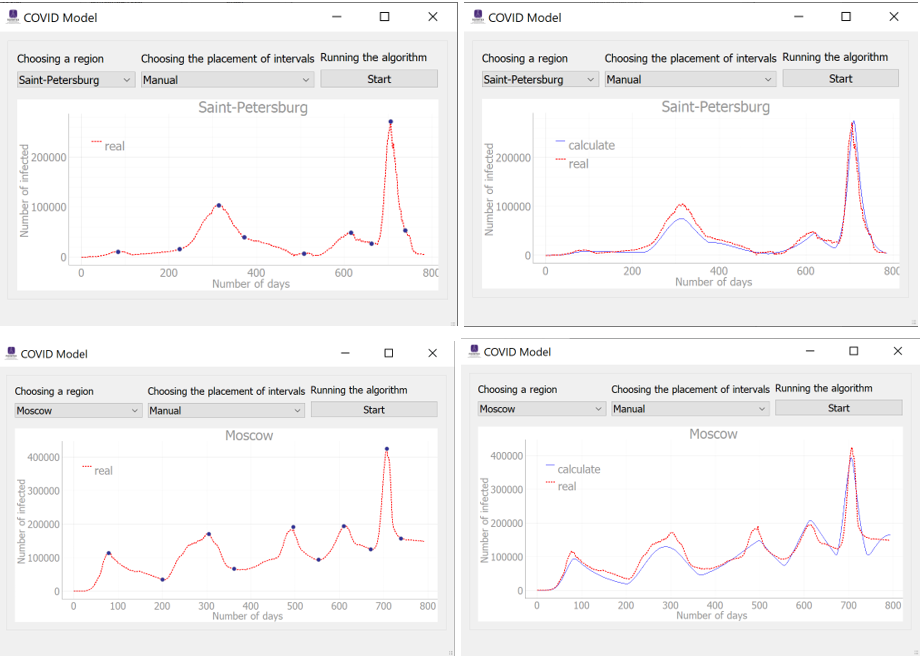Predicting the spread of infectious diseases is an urgent task, since it allows for an assessment of the current situation and making informed decisions on the disease stemming measures to be taken. However, predictive models need constant adjustment and validation of the data obtained according to current data on infection spread dynamics. The present research aims to select and integrate a calibration method for the epidemiological Kermak-McKendrick SEIR model with additional factors. This paper provides an overview and analysis of calibration algorithms for the required parameters of the epidemiological model, as well as numerical experiments comparing the accuracy of the results. The resulting calibration method is the least squares method, since it allows considering boundary values and searching for a local minimum, spending the least amount of time compared to other algorithms.Automatic calibration of the model parameters allows for up-to-date predictions on the spread of infectious diseases with minimal time resources in response to changes in disease data and various quarantine measures. The developed solution can be tailored to other infection spread models.

Automatic calibration; Digital modelling; Digital technologies; Infection spread model; Model prediction

Introduction

The outbreak of the COVID-19 pandemic, which turned out to be one of the deadliest pandemics in history, caused the development of new tools to control the spread of infectious diseases (Berawi et al., 2020). As part of stemming the spread and struggling with the consequences of COVID-19, various predictive models have been developed as a tool for making informed decisions at the governmental level (Zubkova & Efremova, 2022).

The peculiarity of modeling sociotechnical systems is an increase in complexity and a decrease in the level of determinism in comparison with the modeling of technical systems. The lack of adequate management methods for sociotechnical systems leads to suboptimal management decisions, which significantly reduces the quality of management and the efficiency of the system.

Predictive modeling allows revealing stable trends or, conversely, significant changes in socio-economic processes, assessing their probability for the future planning period, identifying possible alternatives, accumulating scientific and empirical material for a reasonable choice of a particular development   concept or a planned solution (Antohonova, 2022; Narayan et al., 2021; Bol et al., 2021). The predictive method is a set of techniques that make it possible to derive propositions based on a certain reliability of the relative future development of the predictive object. Such propositions are based on data analysis, the exogenous and endogenous connections of an object, and their measurement (Antohonova, 2022). Therefore, predicting the consequences of a pandemic allows researchers to determine how various measures of influence on the system will affect the morbidity dynamics (Borovkov et al., 2022). This result is important for making managerial decisions to counteract the COVID-19 spread in various regions at the governmental level (Borovkov et al., 2022; Berawi, 2021).

However, constant additions to the model of infectious diseases, considering the dynamics of quarantine measures and their diversity, as well as the virus evolution, require constant modification, evaluation, and model parameter adjustment due to the variability of miscellaneous factors affecting the result (Pesaran & Yang, 2022). In this respect, in order for the predictive model to remain reliable, it is necessary to compare the actual results with the expected or training data and adjust the model parameters. This process is called calibration. Thanks to it, when the indicators change, the model will adjust and give a result close to the real one (Villaverde et al., 2022).
It is worth noting that manual calibration is a demanding and time-consuming process that needs to be automated to reduce resource exploitation. The number of fitting parameters can be more than ten; the amount of data is not regulated. Therefore, manual calibration will have a negative effect in the form of long and inaccurate work. In the case of the infection spread, it is necessary to automate the process of fitting variables, since changes occur regularly based on large amounts of data, numerous factors and indicators (Osborn et al., 2020). In this regard, manual calibration is a suboptimal process that requires constant recalculation of coefficients, which, with an increase in the amount of data and parameters, will increase the operating time.
The present research aims to select and implement an automated calibration method for predicting morbidity dynamics. In this paper, a regression model of time series based on the Kermack-McKendrick SEIR model is used (Borovkov et al., 2022). Besides factors accepted in the SEIR model, the considered model considers new conditions, with the help of which it was possible to track the dynamics of the COVID-19 outbreak more reliably and obtain a more plausible prediction. In this paper, the calibration method is selected from the available software tools of the Python programming language, and its implementation and integration into the software for predicting the morbidity dynamics during the COVID-19 pandemic are carried out. The main advantages of the Python programming language are accessibility, simplicity, and ease of use and learning, as well as its many libraries and frameworks. The software is verified using publicly available data, and the values obtained are compared with those available based on statistical criteria. The result allows obtaining more accurate estimates of patients, which reduces the time spent on calculations and model adequacy assessments.

Experimental Methods

2.1.  Model description

The paper deals with the analysis and implementation of tools for automated calibration carried out based on a SIR model variant, considering additional factors (vaccination, including revaccination; recurrent morbidity; individual isolation) – Kermak-McKendrick SEIR model (Borovkov et al., 2022). The mathematical interpretation of the model is simultaneous homogeneous differential equations (a time series model). The general scheme of the modified COVID-19 distribution model is shown in Figure 1.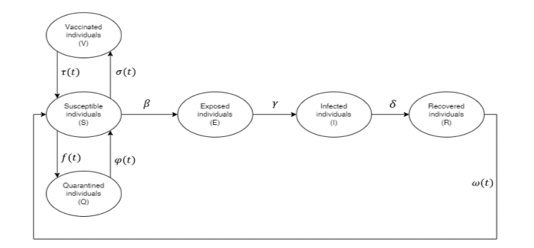Figure 1 General scheme of the modified COVID-19 propagation model (Borovkov et al., 2022; Borovkov et al., 2020)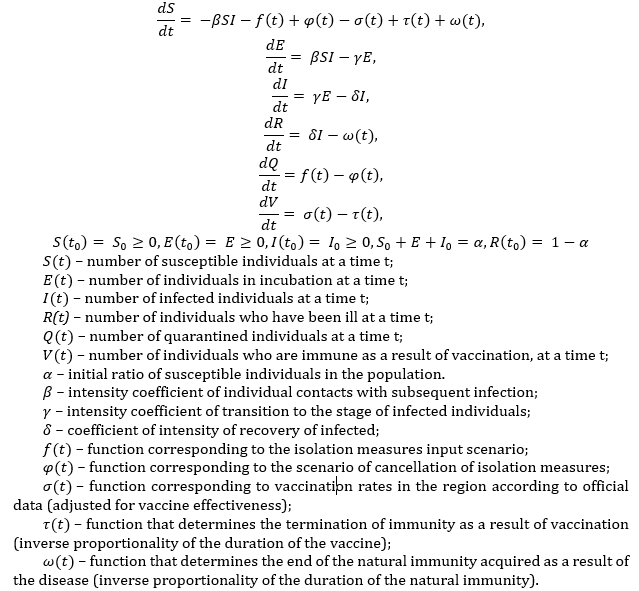The model has a  parameter that represents the intensity of effective contacts, characterizing the infection transmission rate (Borovkov et al., 2020). The effective contact intensity value is a time-dependent function (1):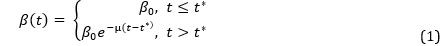The intensity indicator depends not only on the properties of the infection but also on the society’s structure, population density, and a large number of other factors that make up the daily routine of a modern person (Popkov et al., 2022).  The exponent indicator takes into account the gradual increase in the impact of time-control measures introduced since the morbidity level and the effectiveness of control measures . Thus, the pandemic progress over time can be analyzed, and the effectiveness of the stemming measures can be evaluated (Popkov et al., 2022).

The use of the effective contact rate variable  makes it possible to increase the plausibility of the COVID-19 spread dynamics simulation and obtain a more accurate expected morbidity rate. Therefore, this paper considers changes in the  and  parameters depending on the introduced measures of morbidity control and the time that has passed since then.

After developing a predictive model, it is required to validate the obtained results to find out how close they are to the expected ones. To do this, the following properties are checked: adequacy and stability (Maksimej et al., 2014). The adequacy assessment reflects the correspondence of the obtained and source data, and the stability assessment ensures that the model behaves correctly over the entire range.

Thus, if the resulting model does not meet the standards, then it needs to be corrected. This procedure is called calibration. It is iterative. Calibration verifies the reliability of the model results obtained and finds a more accurate solution that is optimally close to real data.

The calibration process consists of 3 stages (Villaverde et al., 2022; Borzooei et al., 2019):

1.   Comparing output results (the adequacy of the model is evaluated);

2.   Balancing the model (the stability of the model is evaluated);

3.   Optimizing the model (parameters are adjusted to improve the output data quality and ensure the required accuracy).

To carry out calibration when predicting the considered model, it is necessary to divide the available data into intervals (waves) and on each of them select the best values of the  and  parameters, based on which the prediction of all infected is made later within the selected time interval.

2.2. Software tools for the calibration method

Software implementation of optimization methods and function fitting to data is present in some Python libraries. In the selected programming environment, there are several possible options for calibration methods:

·      The scipy library, which contains the scipy.optimize.curve_fit() function (SciPy Documentation, 2022a).

·      The lmfit library, which contains the lmfit.minimize() function (LMFIT, 2021)

Both scipy.optimize.curve_fit() and lmfit.minimize() are wrappers for scipy.optimize.leastsq(). Both of these functions offer many advantages over scipy.optimize.leastsq(), however, lmfit.minimize() requires more resources to adjust the input parameters of the function than scipy.optimize.curve_fit().

The main difference between these functions is that lmfit has a Model class, which provides a more flexible and convenient approach to the curve matching task. Moreover, lmfit has a Parameters class, which makes it possible to separately set the parameters and their properties necessary for fitting. In addition, lmfit has the fit_report() method, which allows getting information about the best values of parameters, their standard errors, and other evaluation criteria. The lmfit.minimize() function is more extensive and time-consuming from the perspective of execution steps however, it is more difficult to introduce changes to the scipy.optimize.curve_fit() method in terms of abstraction and structure.

In addition, the scipy.optimize.curve_fit() method implements minimization using the least-squares method, while in the lmfit.minimize() function, there is an option to select the method by which the optimization will be performed.

The brute, basinhopping, dual annealing, differential evolution, and shgo methods are applied to search for a global extremum, other algorithms are applied to search for a local one. For the above problem, it is required to find local minima at all intervals of the graph; therefore, global optimization methods are inappropriate for solving it.

Further, the newton, trust-ncg, trust-exact, trust-krylov, and dogleg methods require a Jacobi matrix and a Hesse matrix to search for a local extremum without considering constraints. Defining a function to calculate the Jacobian and Hessian is demanding, and their execution requires additional program time. Therefore, these functions were not considered for fitting variables, given that these algorithms do not consider constraints. Table 1 shows the differences between appropriate minimization methods.

Table 1 Number of receptors in each container

 Minimization algorithm lmfit.minimize() Features of the minimization algorithm leastsq Minimization of the sum of simultaneous equations squares using a loss function based on the Levenberg-Marquardt algorithm. This method is used if constraints are not needed, because it does not handle boundaries. least_squares Minimization of the sum of simultaneous equations squares using a loss function based on the Levenberg-Marquardt algorithm, using the Trust Region Reflective method. Takes into account constraints (boundary values). This method is an extended version of the leastsq algorithm. nelder Minimization of the scalar function of one or more variables using the Nelder-Mead algorithm. bfgs Minimization of the scalar function of one or more variables using the BFGS algorithm. lbfgsb Minimization of the scalar function of one or more variables using the L-BFGS-B algorithm. A method implemented with reduced memory consumption due to partial loading of vectors from the Hesse matrix. Powell Minimization of the scalar function of one or more variables using the modified Powell algorithm. Cg Minimization of the scalar function of one or more variables using the conjugate gradient algorithm. Cobyla Minimization of the scalar function of one or more variables using the Constrained Optimization by Linear Approximation Algorithm (without gradient calculation). Tnc Minimization of the scalar function of one or more variables using a truncated Newton (TNC) algorithm. This algorithm has a limited number of iterations and is good for nonlinear functions with a large number of independent variables. trust-constr Minimization of the scalar functions is subject to constraints. Slsqp Minimization of the scalar function of one or more variables using Sequential Least Squares Programming (SLSQP) with constraints (The Lagrange-Newton method).

The description of the algorithms is taken from the open documentation of the SciPy library (SciPy Documentation, 2022b).
Within further research, these methods will be verified on common data, that will be taken from an electronic resource on operational data on coronaviruses.  And an analysis will be carried out, and the tested results will be evaluated.

Results and Discussion

3.    Results

When implementing the calibration method, experiments were carried out with several functions:

1.   Personally developed method

2.   scipy.optimize.curve_fit()

3.   lmfit.minimize()

The decision to select the function was based on running each option on the available data from Moscow and St. Petersburg. The criteria considered are the prediction accuracy and the time spent on the execution of the algorithm.

The personally developed method had a standard deviation comparison criterion, but it iterated over the parameter values within the specified boundaries. That is why this method produced the most inaccurate and time-consuming results compared to the others.

The scipy.optimize.curve_fit() method and the lmfit.minimize() method had similar results in time and accuracy, but the final choice depended on the minimization method.

The considered minimization algorithms performed the same task, but in different ways. The leastsq method does not take into account restrictions, which leads to incorrect data on the Moscow graph. When using the trust-constr, tnc, cobyla, cg, bfgs, lbfgsb, and slsqp methods, the parameter values assumed boundary values, which did not correspond to the optimal solution in this section of the curve. In the cases of the nelder and powell methods, the curve fitting process took a long time, but the selected values were not quite accurate. The prediction parameters for the operating time and accuracy changed depending on the various features of the methods.

The results of the algorithms' running time are shown in Table 2.

Table 2 Calculation of the operating time of the program for Saint-Petersburg using the automatic interval selection method

 Method Operation time, min personally method 15,33 scipy.optimize.curve_fit method 3,88 lmfit.minimize method least_squares 1,783 leastsq 1,75 nelder 8,08 powell ?

Thus, the lmfit.minimize() function with the least_squares method was chosen because it provided the most precise indicators that combined the results in terms of operating time, output data reliability, and usability. In addition, the program has developed two approaches for splitting the curve into intervals: manual and automatic. The manual method consists in the user manually splitting the curve into intervals, leaving points in real time. Then the entire segment is divided into the desired intervals using the points that the user has set, and the program begins calibration in each of them. The automatic interval detection method found peaks and troughs independently using scipy.signal methods.find_peaks, scipy.signal.peak_widths, scipy.signal.peak_prominences methods. First, peaks were defined with the scipy.signal method.find_peaks method, after that the search for troughs was carried out. To do this, the length of each peak was determined with the scipy.signal.peak_widths method, and peaks longer than 50 days were selected. Then, in the selected peaks, a height search was performed with the scipy.signal.peak_prominences method and a height selection of more than 10,000 infected. Thus, the selected points were sorted in ascending order, and the curve was divided into intervals, which were then calibrated.

The operation of the program on the Moscow and Saint Petersburg data is shown in Figure 2 and Figure 3, demonstrating the automatic and manual methods, respectively. The data were taken from an open source (Stopcoronavirus.rf, 2022).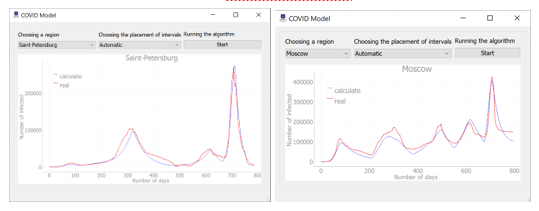Figure 2 The results of the automatic interval selection method on the Moscow and Saint Petersburg data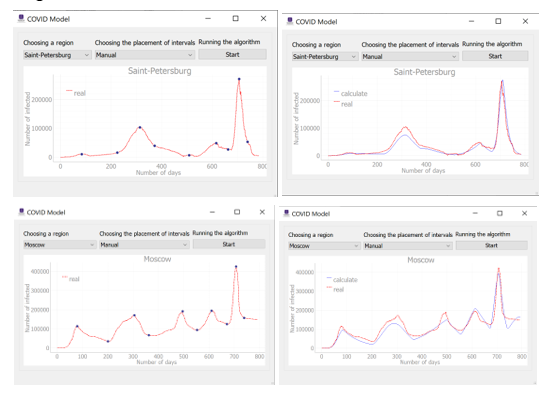Figure 3 The results of the manual interval selection method on the Moscow and Saint Petersburg data

There are plenty of statistical criteria to confirm the data’s reliability. Within the research, the chi-square criteria, the Akaike and Bayes information criterion (Peng et al., 2021; Kalhori et al., 2019), and the difference in areas under the resulting and original graphs were considered.

The values of the criteria for each interval could be obtained during calibration in the form of a report using the lmfit.fit_report() method.

Theoretically, the lower the number of Akaike and Bayes criteria, the more reliable the results. However, throughout the entire fitting, the values of these criteria remain quite large. This is the reason why the resulting graph does not match the original one by 100%. But the obtained values are the most optimal in the current situation. Therefore, with other values of the  and  parameters, information criteria take even more non-zero values. The same applies to the chi-square criterion. In addition, the standard error and confidence interval for each selected value of and  can be observed from the report.

Table 3 demonstrates the average criteria values for all intervals in the case of Saint Petersburg. Values were calculated only for methods that show correct results. The powell algorithm was not considered due to the fact that it takes a long time to calculate parameter values in comparison with the above methods. Table 4 shows the same values for Moscow.

Table 3 Calculation of criteria for Saint Petersburg

 Saint Petersburg Chi average AIC average BIC average least_squares 23456669080.199 1750.138 1754.56 leastsq 221861702553.906 1862.872 1867.295 nelder 23458213317.06 1750.139 1754.562

Table 4 Calculation of criteria for Moscow

 Moscow Chi average AIC average BIC average least_squares 27806867064.608 1378.292 1382.143 leastsq 1245427171708.553 1854.249 1858.885 nelder 27806876506.776 1378.292 1382.143

Table 3 and Table 4 show that the criteria values for least_squares and nelder algorithms differ in the 5th or 7th order in the case of the chi-square criterion, and differ in digits after the decimal point in case of AIC and BIC criteria. Despite the small difference in errors, the least_squares method operates better since it requires less time for calculations.

Further, the area under the graph was checked with the numpy.trapz() function. Table 5 shows the results.

Table 5 Area calculation of the graphs under consideration

 Area difference (number of infected) Area with source data (number of infected) Area with the obtained data (number of infected) Saint Petersburg 3 million 27 million 24 million Moscow 6 million 88 million 82 million

Thus, in Saint Petersburg, the model for predicting the infection spread dynamics has an error of 11.1% of the source data. On the other hand, in Moscow, the model gives an error of 6.8%. Given that the errors in both cases are close to 10%, it can be assumed that the predictive model is adequate, and the prediction results meet expectations.

The discrepancy between the results and the initial data is explained by the fact that the forecasting methods and the available models are imperfect since they cannot fully consider all the factors affecting the real situation. Therefore, these studies are of particular interest among the scientific community, and the developed models are being improved and complicated, which provides more reliable and accurate forecasts.

4.    Discussion

The chosen automatic calibration method showed an adequate result, in which there is a correlation between reliability and the algorithm's operating time. Numerically, by the values of the difference in the areas under the graphs, and graphically, by the illustrations in Figure 2 and Figure 3, it can be seen that the deviations of the prediction results from the actual data are present and have sufficiently high indicators. However, the above solution is optimal, satisfying the needs in terms of both operating time and modeling accuracy. Moreover, considering the result as a whole, it can be noticed that the resulting graph repeats the shape and main waves (peaks and troughs) of the original data and therefore meets the necessary requirements.

It was found that if there are more intervals, then prediction becomes more accurate, but the operating time increases. Due to the frequency of the intervals, their size decreases, so the adjustment of the  and  parameters is carried out at closer numerical values. Thus, there will be fewer outliers and more acceptable values of  and , and, as a result, a more accurate and reliable prediction.

The best result was obtained with the least squares method (LSM). LSM is used to solve various tasks where it is required to minimize the discrepancy (error). Furthermore, this method is successfully applied for finding solutions to nonlinear simultaneous equations and data approximation (Benzerrouk et al., 2013). In the present case, the SIR model variation was used, which constitutes simultaneous ordinary differential equations (SODE) (Borovkov et al., 2022). Therefore, the least squares method is appropriate for solving the problem of minimizing the sum of squared errors between the infected agents in the evaluated model and the current values (Parhusip et al., 2022; Qian, 2022; Smit et al., 2022; Ji et al., 2021).

To adapt the calibration method to other models, the error function should be changed. This function compares the value was calculated by the formulas of the Kermack-McKendrick SEIR model and the reference value. Accordingly, the purpose of calibration is to minimize errors in the entered parameters. The formula for calculating the error is given below (2).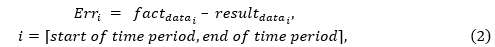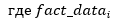– the initial data at the current step of the algorithm,  – the data calculated at the current step of the algorithm with the current selected parameters.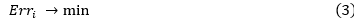Then it is possible to adapt the calibration to other needs: it is necessary to replace the existing formula with any other formula that is required to solve the task, change the reference values, and enter the necessary parameters. Thus, it is necessary to change the original values of  and replace the formula for calculating the current value of . Also, replace the parameters in the Parameter class with those that need to be calibrated.
It is also possible to use this model within a different geographical location, for example, by changing the input data and parameters to those defined in another country (the dynamics of cases, recovered, vaccinated, and other indicators). To do this, you will need to generate all the necessary information in a json file and add it to the file containing the source data.

Conclusion

Within the research, the calibration method was selected and integrated into the predictive epidemiological Kermak-McKendrick SEIR model. For each wave, the values of the calibrated parameters changed, so the method of automatic and manual (graphical) selection of patient waves was implemented. To implement the functions, the Python programming language, and the minimization method from the lmfit library were chosen for model calibration. It is the most abstract, so it allows changing parameters in a more convenient manner and getting estimates of the fit. To minimize the error between the obtained and source data, the least-squares algorithm was selected in the minimization method. It allows considering the boundary values and demonstrates a solid performance in a short period of time. The developed calibration program of the obtained data allows using software to correctly assess the impact of the virus spread stemming measures during a pandemic on the morbidity dynamics. The developed solution can be tailored to other infection spread models.

Acknowledgement

The research is funded by the Ministry of Science and Higher Education of the Russian Federation (contract No. 075-03-2022-010 dated 14.01.2022).

Antohonova, I., 2022. Metody Prognozirovaniya Social'no-Ekonomicheskih Processov. Litres (Forecasting Methods Socially-Economically Processor. Litres.). Russia: Yurait Publishing House

Benzerrouk H., Nebylov A., Salhi H., 2013. Contribution in Information Signal Processing for Solving State Space Nonlinear Estimation Problems. Journal of Signal and Information Processing, Volume 4(4), p. 375

Berawi, M.A., 2021. World Agenda on Sustainable Recovery from the Covid-19 Pandemic: Recover Together, Recover Stronger. International journal of technology, Volume 12(4). p. 671675

Berawi, M.A., Suwartha, N., Kusrini, E., Yuwono, A.H., Harwahyu, R., Setiawan, E.A., Yatmo, Y.A., Atmodiwirjo, P., Zagloel, Y.T., Suryanegara, M., Putra, N., Budiyanto, M.A., Whulanza, Y., 2020. Tackling the COVID-19 Pandemic: Managing the Cause, Spread, and Impact. International Journal of Technology, Volume 11(2), pp. 209214

Bol, D., Giani, M., Blais, A., Loewen, P. J. 2021. The Effect of COVID?19 Lockdowns on Political Support: Some Good News for Democracy? European Journal of Political Research, Volume 60(2), pp. 497–505

Borovkov, A.I., Bolsunovskaya, M.V., Gintciak, A.M., 2022. Intelligent Data Analysis for Infection Spread Prediction. Sustainability, Volume 14(4), p. 1995.

Borovkov, A.I., Bolsunovskaya, M.V., Gintciak, A.M., Kudryavtseva, T.J., 2020. Simulation Modelling Application for Balancing Epidemic and Economic Crisis in the Region. International Journal of Technology, Volume 11(8), p. 15791588

Borzooei, S., Amerlinck, Y., Abolfathi, S., Panepinto, D., Nopens, I., Lorenzi, E., Meucci, L., Zanetti, M.C., 2019. Data Scarcity in Modelling and Simulation of a Large-Scale WWTP: Stop Sign or a Challenge. Journal of Water Process Engineering, Volume 28, pp. 10–20

Ji, Q., Zhao, X., Ma, H., Liu, Q., Liu, Y., Guan, Q., 2021. Estimation of COVID-19 Transmission and Advice on Public Health Interventions. Mathematics, Volume 9(22), p. 2849.

Kalhori, S.N., Ghazisaeedi, M., Azizi, R., Naserpour, A., 2019. Studying The Influence of Mass Media and Environmental Factors on Influenza Virus Transmission in The US Midwest. Public Health, Volume 170, pp. 17–22

LMFIT, 2021. Non-Linear Least-Squares Minimization and Curve-Fitting for Python. Available online at  https://lmfit.github.io/lmfit-py/, Accessed on September 26, 2022

Maksimej, I.V., Smorodin, V.S., Demidenko, O.M., 2014. Razrabotka Imitacionnyh Modelej Slozhnyh Tekhnicheskih Sistem (Development of Simulation Models of Complex Technical Systems). Ministry of Education of the Republic of Belarus, Gomel State University named after F. Skorina

Narayan, P.K., Phan, D.H.B., Liu, G., 2021. COVID-19 Lockdowns, Stimulus Packages, Travel Bans, and Stock Returns. Finance Research Letters, Volume 38, p. 101732

Osborn, J., Berman, S., Bender-Bier, S., D’Souza, G., Myers, M., 2020. Retrospective Analysis of Interventions to Epidemics using Dynamic Simulation of Population Behavior Mathematical Biosciences, Volume 341, p. 108712

Parhusip, H.A., Trihandaru, S., Wicaksono, B.A.A., Indrajaya, D., Sardjono, Y., Vyas, O.P., 2022. Susceptible Vaccine Infected Removed (SVIR) Model for COVID-19 Cases in Indonesia. Science and Technology Indonesia, Volume 7(3), pp. 400–408

Peng, Z., Ao, S., Liu, L., Bao, S., Hu, T., Wu, H., Wang, R., 2021. Estimating Unreported COVID-19 Cases with a Time-Varying SIR Regression Model. International Journal of Environmental Research and Public Health, Volume 18(3), p. 1090

Pesaran, M.H., Yang, C.F., 2022. Matching Theory and Evidence on Covid?19 using a Stochastic Network SIR Model. Journal of Applied Econometrics, Volume 37(6), p. 2904

Popkov, A., Dubnov, Y., Popkov, Y., 2022. Randomized Machine Learning and Forecasting of Nonlinear Dynamic Models Applied to SIR Epidemiological Model. Informatics and Automation, Volume 21(4), pp. 659–677

Qian, Y., 2022. A Non-autonom SIR Model in Epidemiology. In: Artificial Intelligence and Security, X. Sun, X. Zhang, Z. Xia, E. Bertino (ed.), Springer International Publishing, Cham, Switzerland, pp. 230–238

SciPy Documentation, 2022a. SciPy Documentation. Available online at https://docs.scipy.org/doc/scipy/ reference/generated/scipy.optimize.curve_fit.html, Accessed on September 26, 2022

SciPy Documentation, 2022b. SciPy Documentation. Available online at https://docs.scipy.org/doc/scipy /reference/generated/scipy.optimize.curve_fit.html, Accessed on September 26, 2022

Smit, J. M., Krijthe, J. H., Endeman, H., Tintu, A.N., de Rijke, Y.B., Gommers, D.A.M. P.J, Cremer, O.L., Bosman, R.J, Rigter, S., Wils, E.-J., Fenzel, T, Dongelmans, D.A., De-Jong, R., Peters, M.A.A., Kamps, M.J.A., Ramnarain, D., Nowitzky, R., Noteboom, F.G.C.A., De Ruijter, W., Urlings-Strop, LC., Reindersb, M.J.T., 2022. Dynamic Prediction of Mortality In COVID-19 Patients In The Intensive Care Unit: A Retrospective Multi-Center Cohort Study. Intelligence-Based Medicine, Volume 6, p. 100071

Stopcoronavirus.rf, 2022. Stopcoronavirus.rf. Available online at https://xn--80aesfpebagmfblc0a.xn--p1ai/information/, Accessed on September 26, 2022

Villaverde, A. F., Pathirana, D., Fröhlich, F., Hasenauer, J., Banga, J. R., 2022. A Protocol for Dynamic Model Calibration. Briefings in Bioinformatics, Volume 23(1), p. bbab387

Zubkova, D.A., Efremova, M.O., 2022. Analysis of The Intrenational Trends in Modeling the Spread of Infectious Diseases.  Ekonomika i Industriya 5.0 v usloviyah novoj real'nosti (INPROM-2022), pp. 669-672